Impact Factor 4.581 | CiteScore 4.4
More on impact ›

# Frontiers in Environmental Science## ORIGINAL RESEARCH article

Front. Environ. Sci., 09 December 2020 | https://doi.org/10.3389/fenvs.2020.609594

# Experimental Study of Freeze-Thaw/Water Compound Erosion and Hydraulic Conditions as Affected by Thawed Depth on Loessal SlopeWei Wang1,Zhanbin Li1,2,Rui Yang3,Tian Wang1* andPeng Li1,2
• 1State Key Laboratory of Eco-hydraulics in Northwest Arid Region of China, Xi’an University of Technology, Xi’an, China
• 2Key Laboratory of National Forestry Administration on Ecological Hydrology and Disaster Prevention in Arid Regions, Xi’an University of Technology, Xi’an, China
• 3College of Architecture and Civil Engineering, Yan’an University, Yan’an, China

Freeze-thaw cycles have significant influences on slope erosion processes. In this study, simulated rainfall laboratory experiments were implemented to investigate erosion processes and the relationship between the soil loss rate and hydraulics conditions under different thawed depths and rainfall intensities. The results indicated that linear regression could be used to describe the relationship between the soil loss rate and runoff time. Soil loss rate, as measured by the curve slope k (represented the increase rate in the soil loss rate), generally increased with runoff time over different thawed depths across all rainfall intensities. The k values generally increased with rainfall intensity from 0.6 to 1.2 mm/min, with the exception of the 4 cm thawed slope, for which the k values initially increased before decreasing with rainfall intensity from 0.6 to 1.2 mm/min. The mean soil loss rate and range also increased with thawed depth under the same rainfall intensity. Finally, the interaction of rainfall intensity and thawed depth had the greatest effect on soil loss rate, while stream erosion power was the hydraulic parameter that exhibited the best soil loss rate prediction performance. The results presented herein improve the understanding of the response of freeze-thaw/water compound erosion to hydraulic conditions.

## Introduction

Soil erosion is one of the main environmental problems facing the world (Batista et al., 2019; Dazzi and Papa, 2019; Shi et al., 2020), and this is especially true in the Loess Plateau of China (Shi et al., 2019; Guo et al., 2020; Yu et al., 2020; Yue et al., 2020). However, research in this region has focused on hydraulic erosion and wind erosion, while comparatively little is known about freeze-thaw precipitation compound erosion (Xu et al., 2015a, b; Zhang B.J. et al., 2019; Zhang J. et al., 2019; Zhang W. et al., 2019).

Seasonal freeze-thaw (FT) influences soil physical characteristics, such as soil bulk density (Li et al., 2013; Li and Fan, 2014), erodibility (Wang et al., 2017; Cheng et al., 2018), aggregates (Edwards, 2013; Xiao et al., 2019), and water content (Ala et al., 2016; Wang et al., 2019), which make the soil more susceptible to erosion under compound forces (Edwards, 2013; Li and Fan, 2014). As more snow and glaciers melt due to global warming, soil erosion is continually worsening (Ragettli et al., 2015; Wang et al., 2020). Most mid-latitude regions also suffer from seasonal FT erosion (Ragettli et al., 2015; Wang et al., 2019). Depending on Second National Soil Erosion Remote Sensing Survey in China, 13.23% of national territorial area is in danger of FT erosion (Fan et al., 2009). In the loess hilly-gully region of Loess Plateau, there are around 105–125 d with average temperatures below 0°C, and the average annual rainfall is 300–600 mm, which meets the basic conditions necessary for FT erosion (Wang et al., 2019, 2020). Soil erosion during the thawing period is a unique erosion form known as FT-hydraulic compound erosion.

Previous laboratory rainfall experiments on frozen slopes have shown contrasting patterns. Using control FT-water combined erosion experiments, Wang et al. (2019) found that the total sediment yield of a FT slope exhibited increased soil erosion compared to a control slope (Wang et al., 2019). Wang et al. (2020) found that frozen slopes displayed higher sediment yield capacity at equal flow rates when compared with shallow-thawed and unfrozen slopes. However, Zhou et al. (2009) investigated the effects of the thawed depth on black soil erosion under simulated rainfall and found that the erosion amount decreased with increasing thawed depth under lower soil water content. Wei et al. (2015) studied the impacts of FT cycles on runoff and sediment yield of slope land and found that FT cycles greatly increased the sediment yield intensity. In contrast, Ban et al. (2017) found that shallower thawed depths delivered more sediments than deeper thawed depths of high altitude and latitude regions. However, few studies have investigated the effects of thawed depth on soil erosion processes in loess slopes under simulated rainfall.

Generally, hydraulic parameters are essential to describing erosion processes and understanding the hydrodynamic mechanisms of slope erosion (Cuomo et al., 2016; Zi et al., 2016; Xiao et al., 2017; Mirzaee and Ghorbanidashtaki, 2018; Luo et al., 2019). Foster and Meyer (1972) showed that erosion has a positive relationship with shear stress. Govers (1990) found that unit stream power was the best parameter for describing erosion processes. Nearing et al. (1999) and Cai (1995) found stream power to be better than other hydraulic parameters for describing erosion processes. Lu et al. (2009) put forward the stream erosion power concept and established the relationship between soil loss rate and stream erosion power. Wang et al. (2020) found that runoff energy loss could predict thawed soil erosion processes under concentrated flow well. Govers (1992) determined that no existing formula could perform efficiently over the entire range of available data. Soil loss by shallow flows is more closely correlated with flow energy than shear stress (Zhang et al., 2002). Some studies have indicated that stream power is better than shear stress for Dc prediction (Knapen et al., 2007; Cao et al., 2009); however, it is still not clear which parameters are suitable for the prediction of FT precipitation compound erosion under simulated rainfall.

Here, we used laboratory experiments of three rainfall intensities to survey erosion processes and assess the relationship between soil loss rate and various hydraulics parameters under four thawed depths and three gradient rainfall intensities. The specific objectives of this study were to (i) assess erosion processes with runoff time under different thawed depths, and (ii) determine the relationships between soil loss rate and hydraulic parameters.

## Materials and Methods

### Experimental Materials and Design

We used loessal soil collected from the Wangmaogou catchment (37°34′13″–37°36′03″ N and 110°20′26″–110°22′ 46″ E) in Shaanxi Province, China. The collected soil was air-dried and sieved (10 mm) to remove roots, stones, and other debris. The soil particle with 2 mm distribution was then measured using a Mastersizer2000 particle size analyzer (Malvern Instruments, Malvern, United Kingdom), which showed the mechanical composition of the original soil was 0.02 ± 0.003% clay, 65.28 ± 0.43% silt, and 34.7 ± 0.21% sand depending on USDA classification system of soil texture. We then increased the soil water content to 15% by gravity and kept it indoors for 24 h while covered with plastic.

The experimental soil flume was 2 m long, 0.75 m wide, and 0.3 m deep (Figure 1A). The skeleton of the soil flume was made of angle steel, and the wall (0.4 m high) was made from wood to reduce heat exchange so that the soil thawed vertically. The bottom of the flume was filled 0.05 m deep with sand to maintain water permeability. Next, experimental soil was added to a depth of 25 cm in 5 cm layers. Quantitative experimental soil for each layer was obtained depending on the soil dry bulk (1.3 g/cm3), soil water content (15%), and volume of each layer. The surface topography on two sides was raised to an experimental slope of 15°. The area of slope farmland less than 15° accounts for 43.65% of the total area of slope farmland, and the slope gradient 15° is a relative threshold of soil erosion modulus.

FIGURE 1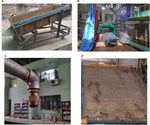Figure 1. The experimental soil flume (A), calibration rainfall intensity (B), rainfall simulator (C) and runoff over the flume (D).

A FT system, 4.5 m long × 2.5 m wide × 2.5 m high, was used to freeze the experimental soil layer. The FT system could produce temperatures of −40 to 30°C with an accuracy of ±1°C. To ensure the soil was completely frozen, the filled soil flume was placed in the FT system for 24 h at −18 to −22°C. We investigated four thawed depths: 0, 2, 4, and 6 cm. The completely frozen soil-filled flume was then placed at room temperature for thawing, during which time a needle was used to measure the thawed depth every 15 min to meet the requirement of thawed depth during the thawing period. The filled soil flume was then divided into 12 grid cells for testing points of thawed depth. We subjected the slope to three rainfall intensities (0.6, 0.9, and 1.2 mm/min) and set a runoff duration of 60 min for each experiment from the rainfall simulator (Figures 1B,C). Each experiment was repeated two times to give a total of 36 experiments and the results were averaged.

The slope was divided into four transects between 0–0.5, 0.5–1.0, 1.0–1.5, and 1.5–2.0 m. The surface flow velocity of the four transects was measured at 1 min intervals using the KMnO4 tracer method (Figure 1D). We multiplied the measured surface flow velocities by a correction factor of 0.65 to obtain the mean flow velocity (Luk and Merz, 1992). The flow widths were measured on the four transects using a ruler at 1-min intervals.

The soil flume bottom was constructed with convergence equipment to collect runoff and sediment at 1 min intervals. The runoff volume was measured in each gathered bucket, and the weight of sediment was recorded after drying in an oven at 105°C for >24 h.

### Methods

The soil loss rate was calculated as the erosion amount per unit area per second:

$S r = e r l ⁢ w ⁢ t (1)$

where Sr is the soil loss rate (g/m2/s); er is the mass of soil loss during the observation time (g); l and w are the length and width of the experimental flume (m), respectively; and t is the observation time (s).

Shear stress, which is related to soil particle detachment and transport, was calculated as follows (Xiao et al., 2017):

$τ = γ m ⁢ g ⁢ R ⁢ J (2)$

where τ is the shear stress (Pa); γm is the muddy water density (kg/m3); g is the gravitational acceleration (m/s2); J is the slope gradient (m/m); and R is the hydraulic radius, which was considered equal to the flow depth under overland flow conditions (m).

It was difficult to directly measure flow depth because the flow depth was shallow and the bed elevation changed with erosion. We calculated flow depth as follows:

$h = Q V ⁢ W ⁢ t (3)$

where h is the flow depth (m); Q is the runoff volume (m3); W is the water width (m); t is the time (s); and V is the mean flow velocity (m/s).

The stream power, which represents power consumed by water flow per unit area, was calculated as previously described (Cao et al., 2009):

$ω = τ ⁢ V ⁢ (4)$

where ω is the stream power (N/m/s); τ is the shear stress (Pa); V is the mean flow velocity (m/s).

The unit stream power, which describes the rate of change of potential energy per unit water mass with time, was calculated as follows (Zhang et al., 2002):

$P = V ⁢ J ⁢ (5)$

where P is the unit stream power (m/s); J is the slope gradient (m/m); V is the mean flow velocity (m/s).

The stream erosion power, which comprehensively expresses the efficiency of runoff erosion and sediment transport, was calculated as described by Lu et al. (2009):

$E = Q m ′ ⁢ H ⁢ (6)$

where E is the stream erosion power [m4/(s⋅m2)]; $Qm′$ is the runoff peak modulus [m3/(s⋅m2)], which is equal to the runoff peak divided by the experimental soil flume area; and H is the mean flow depth (m).

### Data Analysis

The regression analyses and associated figures were drawn using OriginPro 8.5 (OriginLab Inc, Northampton, MA, United States), statistical analyses were conducted with SPSS 16.0 (SPSS Inc, Chicago, IL, United States).

## Results

### Soil Loss Rate Variation With Runoff Time Under Different Thawed Depths

As shown in Figure 2, linear regression was used to describe the relationship between the soil loss rate and runoff time. The determination coefficient (R2) was used to elevate the regression equation (Table 1). Soil loss rate, as measured by the curve slope k (represented the increase rate in the soil loss rate), generally increased with runoff time over different thawed depths across all rainfall intensities (Figure 2 and Table 1), although it plateaued or slightly decreased after 30 min in some trials (e.g., Figure 2C, 4 cm depth).

TABLE 1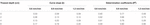Table 1. Regression parameters of erosion processes under different thawed depth and rain intensity.

FIGURE 2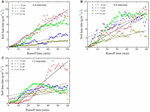Figure 2. The relationship between soil loss rate and runoff time over different thawed depth at 0.6 mm/min (A), 0.9 mm/min (B), and 1.2 mm/min (C).

The R2 values were greater than 0.82 for all tests under 0.6 mm/min intensity. The value of k became greater with deeper thawed depth under 0.6 mm/min intensity and increased to two times when the thawed depth increased from 2 to 4 cm under 0.6 mm/min intensity (Table 1). The value of k was 2.17 times greater at a thawed depth of 4 cm than at 2 cm under 0.6 mm/min intensity (Table 1). As the thawed depth increased from 4 to 6 cm, the value of k increased to 1.31 times under 0.6 mm/min intensity (Table 1).

The R2 values were greater than 0.66 for all tests under 0.9 mm/min intensity (Table 1). The value of k became greater as the thawed depth increased from 0 to 4 cm under 0.9 mm/min intensity (Table 1), while it decreased slightly as the thawed depth increased from 4 to 6 cm under 0.9 mm/min intensity (Table 1). The value of k increased by 1.63 times when the thawed depth increased from 0 to 2 cm under 0.9 mm/min intensity (Table 1), while it was 1.54 times greater at a thawed depth of 4 cm than 2 cm under 0.9 mm/min intensity (Table 1).

The R2 values were 0.56, 0.73 and 0.90 at the thawed depths of 0, 2, and 6 cm under 1.2 mm/min. However, the R2 was 0.26 at a thawed depth of 4 cm under 1.2 mm/min. Except for the thawed depth of 4 cm, the values of k tended to increase as the thawed depth increased from 0 to 6 cm. As the thawed depth increased from 0 to 2 cm, the value of k increased by 1.56 times under 1.2 mm/min intensity (Table 1). Additionally, the value of k was 3.14 times greater at a thawed depth of 6 cm than 2 cm under 1.2 mm/min intensity (Table 1).

For the 0, 2, and 6 cm thawed depths, the values of k increased with increasing rainfall intensity from 0.6 to 1.2 mm/min. The values of k also increased when rainfall intensity increased from 0.6 to 0.9 mm/min for the thawed depth of 4 cm. However, the value of k tended to decrease when the rainfall intensity increased from 0.9 to 1.2 mm/min for the thawed depth of 4 cm.

### Statistical Analysis of Soil Loss Rate Under Different Thawed Depths

Figure 3 shows a box plot of soil loss rate under four thawed depths at rainfall intensities of 0.6 mm/min (a), 0.9 mm/min (b), and 1.2 mm/min (c). The data used to create Figure 3 are summarized in Table 2.

TABLE 2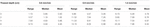Table 2. The statistics of soil loss rate for different thawed depth under changing rainfall intensity.

FIGURE 3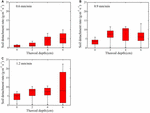Figure 3. The box plot of soil loss rate under different thawed depth at 0.6 mm/min (A), 0.9 mm/min (B), and 1.2 mm/min (C).

The mean soil loss rate increased as thawed depth increased under the same rainfall intensity (Figure 3). A similar phenomenon was also observed for the 1.2 mm/min rainfall intensity except at the thawed depth of 6 cm (Figure 3). At a thawed depth of 0 and 6 cm, the mean soil loss rate increased as rainfall intensity increased. At thawed depths of 2 and 4 cm, the mean soil loss rate increased when the rainfall intensity increased from 0.6 to 0.9 mm/min (Figure 3 and Table 3). However, the mean soil loss rate decreased when the rainfall intensity increased from 0.9 to 1.2 mm/min (Figure 3 and Table 2).

TABLE 3

As shown in Figure 3, the median values increased as the thawed depth increased under rainfall intensities of 0.6 and 0.9 mm/min (Table 2). For the 1.2 mm/min rainfall intensity, the median values tended to increase as the thawed depth increased from 0 to 4 cm (Table 2). However, decreases in the median value were observed at a rainfall intensity of 0.9 mm/min when the thawed depth was from 4 to 6 cm (Table 2). Moreover, the median value increased with increasing rainfall intensity at thawed depths of 0, 2, and 6 cm (Table 2). For a thawed depth of 4 cm, the median value increased as rainfall intensity increased from 0.6 to 0.9 mm/min (Table 2). However, the median value was lower under 0.9 mm/min than under 1.2 mm/min (Table 2).

The range was used to describe the variation in soil loss rate for individual groups (Table 2). The range increased as thawed depth increased under the same rainfall intensity (Table 2). For thawed depths of 0 and 6 cm, the range values also increased as rainfall intensity increased from 0.6 to 1.2 mm/min (Table 2). For thawed depths of 2 and 4 cm, the ranges also increased as rainfall intensity increased from 0.6 to 0.9 mm/min (Table 2); however, the ranges decreased as rainfall intensity increased from 0.9 to 1.2 mm/min (Table 2).

### Relationships Between Soil Loss Rate and Hydraulic Conditions

Figure 4 shows the relationship between soil loss rate and shear stress (a), mean stream power (b), mean unit stream power (c), and stream erosion power (d). All the mean hydraulics conditions were found to have a positive relationship with soil loss rate (Figure 4).

FIGURE 4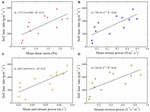Figure 4. The relationships between the soil loss rate and the mean shear stress (A), mean stream power (B), mean unit stream power (C) and stream erosion power (D).

Soil loss rate can be linearly described via mean shear stress (Figure 4A and Table 3). The determination coefficient (R2) is 0.52 and the probabilities test was less than the significance level of 0.01. The erodibility value based on the mean shear stress was 0.17 s/m and the critical shear stress was 0.66 Pa.

The soil loss rate can be fitted to mean stream power with the following power function (Figure 4B and Table 3). The R2 was 0.60 and the probabilities test was less than the significance level of 0.01. The erodibility value based on the mean stream power was 0.78 s2/m2 and the power was 0.61.

The soil loss rate showed a linear increase as the mean unit stream power increased, and the relationship could be fitted with the linear expression (Figure 4C and Table 3). The R2 of Eq. (3) is 0.57 and the probabilities test was less than the significance level of 0.01. The erodibility value based on the mean unit stream power was 0.78 kg/m3 and the critical unit stream power was 0.1 cm/s.

The power function below effectively described the relationship between the soil loss rate and stream erosion power (Figure 4D and Table 3). The R2 value of Eq. (4) was 0.68 and the probabilities test was less than the significance level of 0.01. The erodibility value based on the mean stream power was 0.58 kg/L4 and the power was 0.52.

## Discussion

### The Effect of Rainfall Intensity and Thawed Depth on Soil Loss Rate

Under different rainfall intensities, the k differed with increasing thawed depth. The soil loss rate is determined by hydrodynamic and erosion material conditions. In this study, the hydrodynamic was determined by the rainfall intensity and the soil utilized. For the same rainfall intensity, the k value increased up to a thawed depth of 4 cm. This was likely because shallower thawed soil layers (0 and 2 cm) have slower thawing rates due to heat conduction because the frozen layer will decrease the infiltration ability (Sharratt et al., 2000; Zheng et al., 2001; Wang et al., 2020). As a result, the erodible soil was in short supply, leading to a lower erosion rate. As thawed depth increased (4 and 6 cm), more erodible soil was supplied compared the shallower thawed depths, leading to greater erosion rates. However, the limited hydrodynamic conditions at these depths resulted in decelerating erosion rates. For the 1.2 mm/min rainfall intensity, the erodible soil was still limited for 0 and 2 cm thawed depths. The 4 cm thawed depth showed a unique trend under high intensity rainfall. Specifically, the soil loss rate began to decrease after 30 min of rainfall, which led to the lowest k value in that trial. The soil loss rate decreased because frozen soil melted slowly in the final 30 min of rainfall, but the soil loss rate was still higher than at thawed depths of 0 and 2 cm.

To clarify the correlation of rainfall intensity and thawed depth, the correlations between soil loss rate and rainfall, thawed depth and rainfall intensity-thawed depth were analyzed. As shown in Table 4, soil loss rate was significantly correlated with rainfall intensity, thawed depth, and rainfall intensity-thawed depth in the following order: IH > I > H. These findings indicate that interaction of rainfall intensity and thawed depth has the most important effect on soil loss rate.

TABLE 4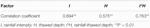Table 4. Correlation coefficients between soil loss rate with coupling effects of rainfall intensity and initial thawed depth.

### Response of Soil Loss Rate to Hydraulics Conditions

In this study, the soil loss rate could be robustly predicted as linear functions of previously identified hydraulic conditions, which is similar to the results of previous studies (Laflen et al., 1991; Nearing et al., 1999; Zhang et al., 2003, 2015). However, we found lower erodibility values than some previous investigations, where erodibility ranged from 8.18 × 10–4 to 8.4 × 10–3 s/m (He et al., 2003; Zhang et al., 2013). Additionally, the critical shear stress was only 10% that obtained from a recent laboratory study (Cao et al., 2009). Zhang et al. (2015) found that the critical shear stress was 12.8 Pa under a steep slope accumulation yield experiment (30∼50°), while we found that critical shear stress was almost 20 times greater than that obtained in their study at 15°. Together, these results suggest that FTed soil has a greater erodibility and is less resistant to erosion (Li and Fan, 2014; Ban et al., 2017; Wang et al., 2020).

In addition, the mean stream power coefficient was larger and the power exponent was smaller than in previous studies (Zhang et al., 2008; Mirzaee and Ghorbanidashtaki, 2018; Wang et al., 2018). The larger coefficient mainly resulted from thawed soil having increased susceptibility to provide sediment sources (Wang et al., 2018, 2020). The smaller power exponent indicated that the increasing amplitude of the loss rate decreased with increased stream power because more energy was used to transport the sediment while the loss energy increased (Zhang et al., 2009; Shen et al., 2016; Xiao et al., 2017).

The erodibility value of unit stream power was larger and the critical unit stream power value was smaller than for previously conducted studies (Wang et al., 2017, 2020). This can be attributed to freeze-thawed soil being more easily eroded (Cuomo et al., 2016; Zi et al., 2016). Many erosion models based on processes utilize stream power to establish transport capacity. In our experiments, stream power could not predict loss rate well, similar to the results of previous studies (Nearing et al., 1999; Zhang et al., 2002, 2003; Wang et al., 2016).

The probabilities test (P < 0.01) indicate that all the hydraulics conditions examined could be used to describe the changes in soil loss rate. However, based on the R2 values, stream erosion power best described the relationship between the soil loss rate and the hydraulic conditions, followed by stream power, unit stream power and shear stress. Flow energy has been shown to be more closely related to soil loss rate than shear stress, as well as to be a useful indicator for describing soil loss rate (Nearing et al., 1999; Zhang et al., 2002; Wang et al., 2016; Xiao et al., 2017). Shear stress is mainly related to slope and flow depth, and thus reflects the response of eroded slope surfaces to concentrated flow. However, water depth is determined by dividing the runoff by the average water width. As a result, the horizontal and vertical evolution of the rill network cause increased inaccuracy of the measured water width. Hence, shear stress was not good predictive parameter in this study. For mean stream power and unit stream power, flow velocity, which was measured by KMnO4 tracking, was the crucial parameter. The flow velocity is the average of different rill velocities resulting from eroded surfaces; thus, flow velocity error increases with rill amount. As a result, hydraulic conditions based on flow velocity will also become less robust. However, the predictive power of soil loss rate from stream or unit stream power was still better than that of shear stress. Finally, stream erosion power is calculated from the flow peak and average flow depth. The average flow depth can be obtained from total runoff amount divided by the underlayer area, which has lower measurement error. Thus, stream erosion power is a better hydraulic parameter for predicting soil loss rate.

## Conclusion

In this study, experimental investigations of erosion processes of different thawed depth loessal slopes were conducted under different rainfall intensities. The results indicated that linear regression could be used to describe the relationship between the soil loss rate and runoff time. The k values increased as rainfall intensity increased from 0.6 to 1.2 mm/min except for the 4 cm thawed depth slope. For the 4 cm thawed depth, the values of k increased first, then decreased as the rainfall intensity increased from 0.6 to 1.2 mm/min. In general, the mean soil loss rate increased with thawed depth under each rainfall intensity. Additionally, the range increased as thawed depth increased under the same rainfall intensity. We observed a significant relationship between soil loss rate and rainfall intensity, thawed depth, and their interaction, and their interaction was found to have the most important effects on soil loss rate. After testing multiple hydraulic conditions, stream erosion power was found to be the best predictor of soil loss rate.

## Data Availability Statement

The raw data supporting the conclusions of this article will be made available by the authors, without undue reservation.

## Author Contributions

WW and TW conceived the main idea of this manuscript. ZL, RY, and PL designed and performed the experiment. WW wrote the manuscript and all authors contributed to improving the manuscript.

## Funding

This research was supported by the National Key Research and Development Program of China (No. 2017YFC0504704), the National Natural Science Foundation of China (52009104 and 51779204), the Project of Ecological Restoration and Environmental Protection in the Upper and Middle Watershed of the Yellow River (QNZX-2019-03), the Shaanxi Province Innovation Talent Promotion Plan Project Technology Innovation Team (Nos. 2018TD-037 and 2020TD-023).

## Conflict of Interest

The authors declare that the research was conducted in the absence of any commercial or financial relationships that could be construed as a potential conflict of interest.

## Acknowledgments

We thank the reviewers for their useful comments and suggestions.

## References

Ala, M., Liu, Y., Wang, A. Z., and Niu, C. Y. (2016). Characteristics of soil freeze-thaw cycles and their effects on water enrichment in the rhizosphere. Geoderma 264, 132–139. doi: 10.1016/j.geoderma.2015.10.008

Ban, Y. Y., Lei, T. W., Chen, C., Yin, Z., and Qian, D. F. (2017). Meltwater erosion process of frozen soil as affected by thawed depth under concentrated flow in high altitude and cold regions. Earth Surf. Process. Landf. 42, 2139–2146. doi: 10.1002/esp.4173

Batista, P. V., Davies, J., Silva, M. L., and Quinton, J. N. (2019). On the evaluation of soil erosion models: are we doing enough? Earth Sci. Rev. 197:102898. doi: 10.1016/j.earscirev.2019.102898

Cai Guoqiang (1995). Small Watershed Runoff Process Model. The Second Session of National Fundamental Theories of Sediment Transport Research Symposium. Beijing: China Water Power Press, 233-238.

Cao, L. X., Zhang, K. L., Dai, H. L., and Guo, Z. L. (2009). Modeling soil detachment on unpaved road surfaces on the loess plateau. Trans. ASABE 54, 1377–1384. doi: 10.13031/2013.39039

Cheng, Y., Li, P., Xu, G., Li, Z., Wang, T., Cheng, S., et al. (2018). The effect of soil water content and erodibility on losses of available nitrogen and phosphorus in simulated freeze-thaw conditions. Catena 166, 21–33. doi: 10.1016/j.catena.2018.03.015

Cuomo, S., Della Sala, M., and Pierri, M. (2016). Experimental evidences and numerical modelling of runoff and soil erosion in flume tests. Catena 147, 61–70. doi: 10.1016/j.catena.2016.06.044

Dazzi, C., and Papa, G. L. (2019). Soil genetic erosion: new conceptual developments in soil security. Int. Soil Water Conserv. Res. 7, 317–324. doi: 10.1016/j.iswcr.2019.08.001

Edwards, L. M. (2013). The effects of soil freeze—thaw on soil aggregate breakdown and concomitant sediment flow in Prince Edward Island: a review. Can. J. Soil Sci. 93, 459–472. doi: 10.4141/cjss2012-059

Fan, H. M., Zhang, R. F., Zhou, L. L., Wu, M., and Liu, Y. H. (2009). Impact of climate change on freeze-thaw function and freeze-thaw erosion in black soil region of Northeast of China. J. Arid Land Res. Environ. 23, 48–53.

Foster, G. R., and Meyer, L. D. (1972). Transport of soil particles by shallow flow. Trans. ASAE 15, 99–102. doi: 10.13031/2013.37840

Govers, G. (1990). Empirical relationships for the transport capacity of overland flow: erosion, transport, and deposition process. LAHS Publ. 1, 45–63.

Govers, G. (1992). Relationship between discharge, velocity, and flow area for rills eroding loose, non–layered materials. Earth Surf. Process. Landf. 17, 515–528. doi: 10.1002/esp.3290170510

Guo, M., Wang, W., Li, J., Bai, Y., Kang, H., and Yang, B. (2020). Runoff characteristics and soil erosion dynamic processes on four typical engineered landforms of coalfields: an in-situ simulated rainfall experimental study. Geomorphology 349:106869. doi: 10.1016/j.geomorph.2019.106896

He, X. W., Zhang, G. H., and Liu, B. Y. (2003). Soil detachment by shallow flow on slopes. Trans. CSAE 19, 52–55.

Knapen, A., Poesen, J., and De Baets, S. (2007). Seasonal variations in soil erosion resistance during concentrated flow for a loess-derived soil under two contrasting tillage practices. Soil Tillage Res. 94, 425–440. doi: 10.1016/j.still.2006.09.005

Laflen, J. M., Elliot, W. J., Simanton, J. R., Holzhey, C. S., and Kohl, K. D. (1991). WEPP: soil erodibility experiments for rangeland and cropland soils. J. Soil Water Conserv. 46, 39–44.

Li, G. Y., and Fan, H. M. (2014). Effect of freeze-thaw on water stability of aggregates in a black soil of northeast China. Pedosphere 24, 285–290. doi: 10.1016/S1002-0160(14)60015-1

Li, Q., Liu, G. B., Xu, M. X., Sun, H., Zhang, Z., and Gao, L. Q. (2013). Effect of seasonal freeze-thaw on soil anti-scouribility and its related physical property in hilly loess plateau. Trans. Chin. Soc. Agric. Eng. 29, 105–112.

Lu, K. X., Li, Z. B., and Ju, H. (2009). Application of runoff erosion power in the calculation of soil erosion and sediment yield on hillslopes. J. Water Resour. Water Eng. 20, 70–73.

Luk, S. H., and Merz, W. (1992). Use of the salt tracing technique to determine the velocity of overland flow. Soil Technol. 5, 289–301.

Luo, J., Zheng, Z., Li, T., and He, S. (2019). The changing dynamics of rill erosion on sloping farmland during the different growth stages of a maize crop. Hydrol. Process. 33, 76–85. doi: 10.1002/hyp.13312

Mirzaee, S., and Ghorbanidashtaki, S. (2018). Deriving and evaluating hydraulics and detachment models of rill erosion for some calcareous soils. Catena 164, 107–115. doi: 10.1016/j.catena.2018.01.016

Nearing, M. A., Simanton, J. R., Norton, L. D., Bulygin, S. J., and Stone, J. J. (1999). Soil erosion by surface water flow on a stony, semiarid hillslope. Earth Surf. Process. Landf. 24, 677–686. doi: 10.1002/(SICI)1096-9837(199908)24:8<677::AID-ESP981>3.0.CO;2-1

Ragettli, S., Pellicciotti, F., Immerzeel, W. W., Miles, E. S., Petersen, L., Heynen, M., et al. (2015). Unraveling the hydrology of a Himalayan catchment through integration of high resolution in situ data and remote sensing with an advanced simulation model. Adv. Water Resour. 78, 94–111. doi: 10.1016/j.advwatres.2015.01.013

Sharratt, B. S., Lindstrom, M. J., Benoit, G. R., Young, R. A., and Wilts, A. (2000). Runoff and soil erosion during spring thaw in the northern US Corn Belt. J. Soil Water Conserv. 55, 487–494.

Shen, N., Wang, Z. L., and Wang, S. (2016). Flume experiment to verify WEPP rill erosion equation performances using loess material. J. Soils Sediments 16, 2275–2285. doi: 10.1007/s11368-016-1408-3

Shi, P., Feng, Z. H., Gao, H. D., Li, P., Zhang, X. M., Zhu, T. T., et al. (2020). Has “Grain for Green” threaten food security on the Loess Plateau of China? Ecosyst. Health Sustainabil. 6:1709560. doi: 10.1080/20964129.2019.1709560

Shi, P., Zhang, Y., Li, P., Li, Z. B., Yu, K. X., Ren, Z. P., et al. (2019). Distribution of soil organic carbon impacted by land-use changes in a hilly watershed of the Loess Plateau, China. Sci. Total Environ. 652, 505–512. doi: 10.1016/j.scitotenv.2018.10.172

Wang, D. D., Wang, Z. L., Shen, N., and Chen, H. (2016). Modeling soil detachment capacity by rill flow using hydraulic parameters. J. Hydrol. 535, 437–479. doi: 10.1016/j.jhydrol.2016.02.013

Wang, T., Li, P., Hou, J., Li, Z., Ren, Z., Cheng, S., et al. (2018). Response of the meltwater erosion to runoff energy consumption on loessal slopes. Water 10:1522. doi: 10.3390/w10111522

Wang, T., Li, P., Li, Z., Hou, J., Xiao, L., Ren, Z., et al. (2019). The effects of freeze–thaw process on soil water migration in dam and slope farmland on the Loess Plateau, China. Sci. Total Environ. 666, 721–730. doi: 10.1016/j.scitotenv.2019.02.284

Wang, T., Li, P., Liu, Y., Hou, J., Li, Z., Ren, Z., et al. (2020). Experimental investigation of freeze-thaw meltwater compound erosion and runoff energy consumption on loessal slopes. Catena 185:104310. doi: 10.1016/j.catena.2019.104310

Wang, T., Li, P., Ren, Z., Xu, G., Li, Z., Yang, Y., et al. (2017). Effects of freeze-thaw on soil erosion processes and sediment selectivity under simulated rainfall. J. Arid Land 9, 234–243. doi: 10.1007/s40333-017-0009-3

Wei, X., Li, X. G., and Huang, C. H. (2015). Impacts of freeze-thaw cycles on runoff and sediment yield of slope land. Trans. Chin. Soc. Agric. Eng. 31, 157–163.

Xiao, H., Liu, G., Liu, P., Zheng, F., Zhang, J., and Hu, F. (2017). Response of soil erosion rate to the hydraulic conditions of concentrated flow on steep loessial slopes on the Loess Plateau of China. Hydrol. Process. 31, 2613–2621. doi: 10.1002/hyp.11210

Xiao, L., Zhang, Y., Li, P., Xu, G., Shi, P., and Zhang, Y. (2019). Effects of freeze-thaw cycles on aggregate-associated organic carbon and glomalin-related soil protein in natural succession grassland and Chinese pine forest on the Loess Plateau. Geoderma 334, 1–8. doi: 10.1016/j.geoderma.2018.07.043

Xu, X., Liu, Z. Y., Xiao, P. Q., Guo, W., Zhang, H., Zhao, C., et al. (2015a). Gravity erosion on the steep loess slope: behavior, trigger and sensitivity. Catena 135, 231–239. doi: 10.1016/j.catena.2015.08.005

Xu, X., Zhang, H., Wang, W., Zhao, C., and Yan, Q. (2015b). Quantitative monitoring of gravity erosion using a novel 3D surface measuring technique: validation and case study. Nat. Hazards 75, 1927–1939. doi: 10.1007/s11069-014-1405-z

Yu, Y., Zhao, W., Martinez-Murillo, J., and Pereira, P. (2020). Loess Plateau: from degradation to restoration. Sci. Total Environ. 738:140206. doi: 10.1016/j.scitotenv.2020.140206

Yue, L., Juying, J., Bingzhe, T., Binting, C., and Hang, L. (2020). Response of runoff and soil erosion to erosive rainstorm events and vegetation restoration on abandoned slope farmland in the Loess Plateau region, China. J. Hydrol. 584:124694. doi: 10.1016/j.jhydrol.2020.124694

Zhang, B. J., Zhang, G., Yang, H., and Wang, H. (2019). Soil resistance to flowing water erosion of seven typical plant communities on steep gully slopes on the Loess Plateau of China. Catena 173, 375–383. doi: 10.1016/j.catena.2018.10.036

Zhang, G. H., Liu, B. Y., Nearing, M. A., Huang, C. H., and Zhang, K. L. (2002). Soil detachment by shallow flow. Trans. ASABE 45, 351–357. doi: 10.13031/2013.8527

Zhang, J., Yang, M., Deng, X., Liu, Z., and Zhang, F. (2019). The effects of tillage on sheet erosion on sloping fields in the wind-water erosion crisscross region of the Chinese loess plateau. Soil Tillage Res. 187, 235–245. doi: 10.1016/j.still.2018.12.014

Zhang, W., Xing, S., and Hou, X. (2019). Evaluation of soil erosion and ecological rehabilitation in Loess Plateau region in Northwest China using plutonium isotopes. Soil Tillage Res. 191, 162–170. doi: 10.1016/j.still.2019.04.004

Zhang, G. H., Liu, B. Y., Liu, G. B., He, X. W., and Nearing, M. A. (2003). Detachment of undisturbed soil by shallow flow. Soil Sci. Soc. Am. J. 67, 713–719. doi: 10.2136/sssaj2003.7130

Zhang, G. H., Liu, G. B., Tang, K. M., and Zhang, X. C. (2008). Flow detachment of soils under different land uses in the Loess Plateau of China. Trans. Am. Soc. Agric. Biol. Eng. 51, 883–890. doi: 10.13031/2013.24527

Zhang, G. H., Liu, Y. M., Han, Y. F., and Zhang, X. C. (2009). Sediment transport and soil detachment on steep slopes: II. Sediment feedback relationship. Soil Sci. Soc. Am. J. 73, 1298–1304. doi: 10.2136/sssaj2009.0074

Zhang, L., Gao, Z., Yang, S., Li, Y., and Tian, H. (2015). Dynamic processes of soil erosion by runoff on engineered landforms derived from expressway construction: a case study of typical steep spoil heap. Catena 128, 108–121. doi: 10.1016/j.catena.2015.01.020

Zhang, Y. D., Wu, S. F., Feng, H., and Huo, Y. Y. (2013). Experimental study of rill dynamic development process and its critical dynamic conditions on loess slope. J. Sediment Res. 2, 25–32.

Zheng, X., Van Liew, M. W., and Flerchinger, G. N. (2001). Experimental study of the infiltration into a bean stubble field during seasonal freeze-thaw period. Soil Sci. 166, 3–10. doi: 10.1097/00010694-200101000-00002

Zhou, L. L., Wang, T. L., Fan, H. M., Wu, M., Chai, Y., Liu, J., et al. (2009). Effects of incompletely thawed layer on black soil slope rainfall erosion. J. Soil Water Conserv. 23, 1–5.

Zi, T., Kumar, M., Kiely, G., Lewis, C., and Albertson, J. (2016). Simulating the spatio-temporal dynamics of soil erosion, deposition, and yield using a coupled sediment dynamic and 3d distributed hydrologic model. Environ. Model. Softw. 83, 310–325. doi: 10.1016/j.envsoft.2016.06.004

Keywords: freeze-thaw, rainfall, thawed depth, compound erosion, hydraulic conditions

Citation: Wang W, Li Z, Yang R, Wang T and Li P (2020) Experimental Study of Freeze-Thaw/Water Compound Erosion and Hydraulic Conditions as Affected by Thawed Depth on Loessal Slope. Front. Environ. Sci. 8:609594. doi: 10.3389/fenvs.2020.609594

Received: 23 September 2020; Accepted: 17 November 2020;
Published: 09 December 2020.

Edited by:

Ataollah Kavian, Sari Agricultural Sciences and Natural Resources University, Iran

Reviewed by:

Bingbing Zhu, Shaanxi Normal University, China
Fengbao Zhang, Northwest A&F University, China

Copyright © 2020 Wang, Li, Yang, Wang and Li. This is an open-access article distributed under the terms of the Creative Commons Attribution License (CC BY). The use, distribution or reproduction in other forums is permitted, provided the original author(s) and the copyright owner(s) are credited and that the original publication in this journal is cited, in accordance with accepted academic practice. No use, distribution or reproduction is permitted which does not comply with these terms.

*Correspondence: Tian Wang, t.wang@xaut.edu.cn